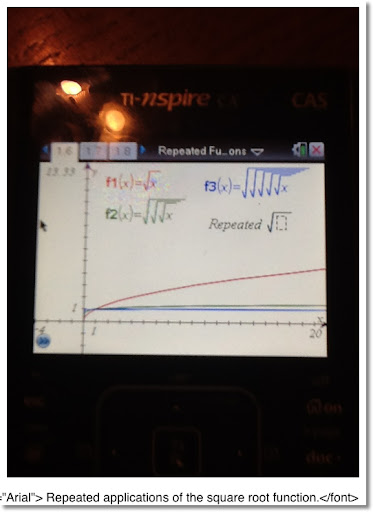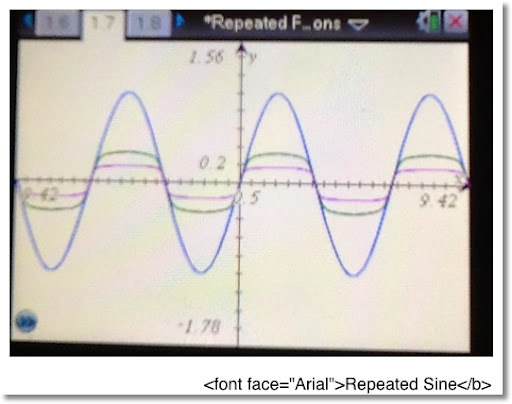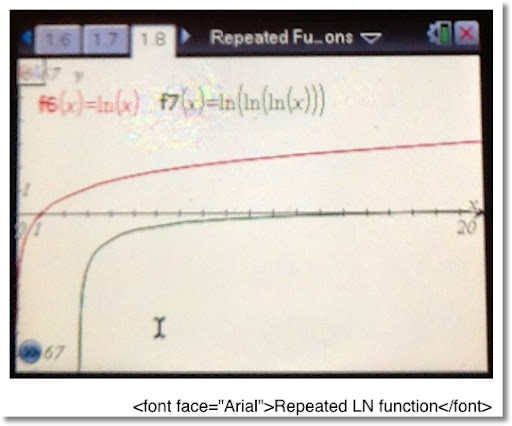## Wednesday, February 8, 2012

### Repeated Applications of Functions

Repeated Functions

If you have ever owned a calculator, chances are you done this: you enter a number and press the square root button many times in a row to see what happens.

For example, you enter say 200. And then you push the square root button. Here are the results for 10 applications of the square root function (to eight decimal places):

14.14213562
3.76060309
1.93922745
1.39256147
1.18006842
1.08630954
1.04226174
1.02091221
1.01040200
1.00518755

Eventually with any positive number, if you press the square root function enough times, you get 1 as a repeated answer.

The following graph shows three functions:

The function in red is the normal square root function.

The function in green shows what happens when the square root function is applied three times to each number.

The function in blue shows what happens when the square root function is applied five times to each number.

Each of the graphs on this blog have been produced using an TI nSpire CAS calculator. All photographs were taken using an iPad 2.Finding a Closed Form for Repeating the Square Root

If we want to describe what a function would look like when applying the square root n times, we can use a nice, closed, and compact formula.

Let f(x) = √ x = x ^(1/2)

Then

f^n(x) = x ^ 1/2 ^ 1/2 ^ .... ^ 1/2 (n times)
= x ^ (1/ (2^n))
= x ^ (2 ^ -n)

Repeated Sine and Logarithm

Now let's apply common scientific functions and repeat them. First the sine function for x ∈ [-3 π, 3 π].The graph in blue represents the normal sine function. (x is in radians)

The graph in green is sine repeated 25 times.

The graph in magenta is sine repeated 100 times.

Note with repeated applications of sine, the graph gets more flatter, towards 0, for each point. Could the approximation formula sin x ≈ x have something to do with this?

This next graph shows what happens when you repeatedly apply the natural logarithmic function:The graph in red is the normal natural logarithmic function.

The graph in green represents the natural logarithm function applied three times.

Something interesting happens with repeating the natural logarithm function. Eventually, you will get a complex number (or an error message on a standard scientific calculator).

Cases in point:

When x=2,
ln 2 = 0.69314718 (to eight decimal places)
ln ln 2 = -0.36651292
ln ln ln 2 = -1.00372150 + π i

When x = 50
ln 50 = 3.91202301 (to eight decimal places)
Repeated applications of the natural logarithm yield:
1.36405463
0.31046161
-1.16969502
0.15674305 + π i

I hope you enjoy this blog, until next time,

Eddie

This blog is property of Edward Shore. © 2012

### Texas Instruments: TI-36X Pro and TI-30X Pro Mathprint

Essentially, the Texas Instruments TI-36X Pro and the TI-30X MathPrint are functionally equivalent.  What makes the two calculators differe...